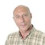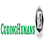Type Here to Get Search Results !

# Analysis of Algorithms - CodingHumans

2

## ANALYSIS OF ALGORITHMS

In computer science, the analysis of algorithms is the process of finding the computational complexity of algorithms – the amount of time, storage, or other resources needed to execute them.

Here we come across following topics:

PROGRAMMING  PERFORMANCE

Performance of a program: The performance of a program  is measured based on  the  amount of computer  memory  and  time   needed to run a program.

The two approaches which are used to measure the performance of the program are:
1. Analytical method called the Performance Analysis.
2. Experimental method  called the Performance Measurement.

SPACE  COMPLEXITY

As said above the space  complexity is one  of the  factor  which  accounts for  the  performance  of the  program. The space  complexity  can be measured using experimental method, which is done by  running  the program and then measuring the actual space occupied by the program during execution. But this is done very rarely. We estimate the space complexity of the program before running the program.

Space complexity is the sum of  the following components :

i) Instruction space:

The program which is written by the  user  is  the  source  program. When this program is compiled, a compiled version of  the  program  is generated. For executing the program an executable  version of the program is  generated. The space  occupied  by  these  three  when  the  program is under execution, will account for the instruction space.

ii) Data space:

The space needed  by  the  constants,  simple  variables,  arrays,  structures and other data structure s will account for the data space.

• Structure size –   It  is  the sum of   the size of component variables of the structure .
• Array size –  Total  size  of  the  array  is  the  product  of  the  size  of the data type and the number of array locations.

The Data space depends on the following factors:

iii) Environment stack space :

The Environment stack  space  is used  for saving  information  needed to resume  execution  of  partially  completed  functions.  That  is  whenever the control of the program is transferred  from  one  function  to  another  during a function call, then the values  of  the  local   variable   of  that  function and return address are stored in the environment stack. This information is retrieved when  the control  comes  back to  the  same function.

The environment stack space depends on the following factors:
2. Values of all local variables and formal parameters .
The Total  space occupied by  the  program during the  execution of the program is the sum of the fixed space and the variable space.

• Fixed space - The space   occupied  by  the  instruction  space,  simple variables and constants.
• Variable space  - The  dynamically allocated  space  to  the various data structures and the environment stack space varies according to the input from the user.

Space complexity S(P) = c + Sp

Here c is a Fixed space  or constant  space Sp is  Variable space

We will  be  interested  in  estimating  only  the  variable  space  because  that is the one which varies according to the user input.

TIME  COMPLEXITY

Time complexity : Time complexity of the program  is defined as the amount of computer time it needs to run to completion

The  time complexity can be  measured,  by  measuring  the  time taken by the program when it is executed.  This  is  an experimental  method. But this is done very rarely. We always try to  estimate  the  time consumed by the program even before it is run for the first time.

The time complexity of the program  depends  on  the  following  factors:

Compiler used –  some compilers  produce optimized  code which consumes less time to get executed.
Compiler options – The optimization options can be set in the options of the compiler.
Target computer  –  The speed  of  the  compute r  or  the  number of instructions  executed  per  second  differs  from one computer to another.

The total time taken for the execution of the program is the sum of the compilation time and the execution time.

(i) Compile time – The  time  taken  for  the  compilation  of  the program  to  produce the  intermediate  object  code  or   the compiler version of the program.  The  compilation  time  is  taken only once as it is enough if the program is compiled  once.  If  optimized  code  is  to  be  genera t e d,  then  the  compilation  time will be higher.

(ii) Run time  or Execution time - The time taken  for  the execution  of the program.  The optimized code  will  take  less time to get executed.

Time complexity T(P) = c + Tp

Here c is a Compile time Tp is a Run  time or  execution time

We will be interested in estimating  only  the  execution  time as this  is  the one which varies according to the user input.

Estimating the Execution time:

Steps in estimating the execution time of program:

(i) Identify one or more key operations and  determine  the  number  of  times these  are perform ed. That  is  find  out  how  many  key  operations are  present  inside  a  loop  and  how  many  times  that loop is executed.
(ii) Determine the total number of steps executed by the program.

The time complexity will be proportional to the sum of the above two.

ASYMPTOTIC  NOTATIONS

Asymptotic notations –  Asymptotic notations are  the  notations used to describe the behavior of the time or space complexity.

Let us represent the time complexity and the space complexity using the common function f(n).

The various asymptotic notations are:

(i) O ( Big Oh notation )
(ii) Ω ( Omega notation )
(iii) θ ( Theta notation )
(iv) o ( Little Oh notation )

O - Big Oh notation

The big Oh notation provides an upper bound for the function f(n).

The  function  f(n)  =  O(g(n))  if  and  only  if  there  exists  positive  constants c and n 0 such that f(n) ≤ cg(n) for all n ≥ n0.

Examples:

1. f(n) = 3n + 2

Let us take g(n) = n
c = 4
n0 = 2

Let us check the above condition

3n + 1 ≤  4n for all n ≥ 2

2. f(n) = 10n^2 + 4n + 2

Let us take g(n) = n 2
c  =  11
n 0  = 6

Let us check the above condition

10n^2 + 4n + 2 ≤ 11n for all n ≥ 6

The  condition is satisfied. Hence f(n) = O(n^2).

Ω - Omega notation

The Ω notation gives the lower bound for the function f(n).

The function  f(n)  =  Ω(g( n))  if  and  only  if  there  exists  positive  constants c and n 0 such that f(n) ≥ cg(n) for all n ≥ n 0.

Examples:

1. f(n) = 3n + 2

Let us take g(n) = n
c = 3
n0 = 0

Let us check the above condition

3n + 1 ≥ 3n for all n ≥ 0

The  condition is satisfied. Hence f(n) = Ω(n).

2. f(n) = 10n^2 + 4n + 2

Let us take g(n) = n^2
c  =  10
n0  = 0

Let us check the above condition

10n^2 + 4n + 2 ≥ 10n  for all n ≥ 0

The condition is satisfied.Hence f(n) =  Ω(n^2 ).

θ -Theta notation

The theta notation is used when the function f(n)  can  be  bounded  by both from above and below the same function g(n).

f(n) = θ(g(n)) if and only if there exists some positive constants c1 and c2
and n0, such that c1g(n) ≤ f(n) ≤ c2g(n) for all n ≥ n0.

We have seen in the previous two cases,

3n + 2 = O(n) and 3n + 2 = Ω(n)

Hence we can write 3n + 2 = θ(n)

o - Little Oh notation

f(n) = o(g(n)) if and only if f(n) = O(g(n)) and f(n) ≠ Ω(g( n))

For example,

3n + 2  =  O(n 2 ) but 3n + 2 ≠ Ω(n^2 )

Therefore it can be written as 3n + 2 = o(n^2)

Tags

1.1.